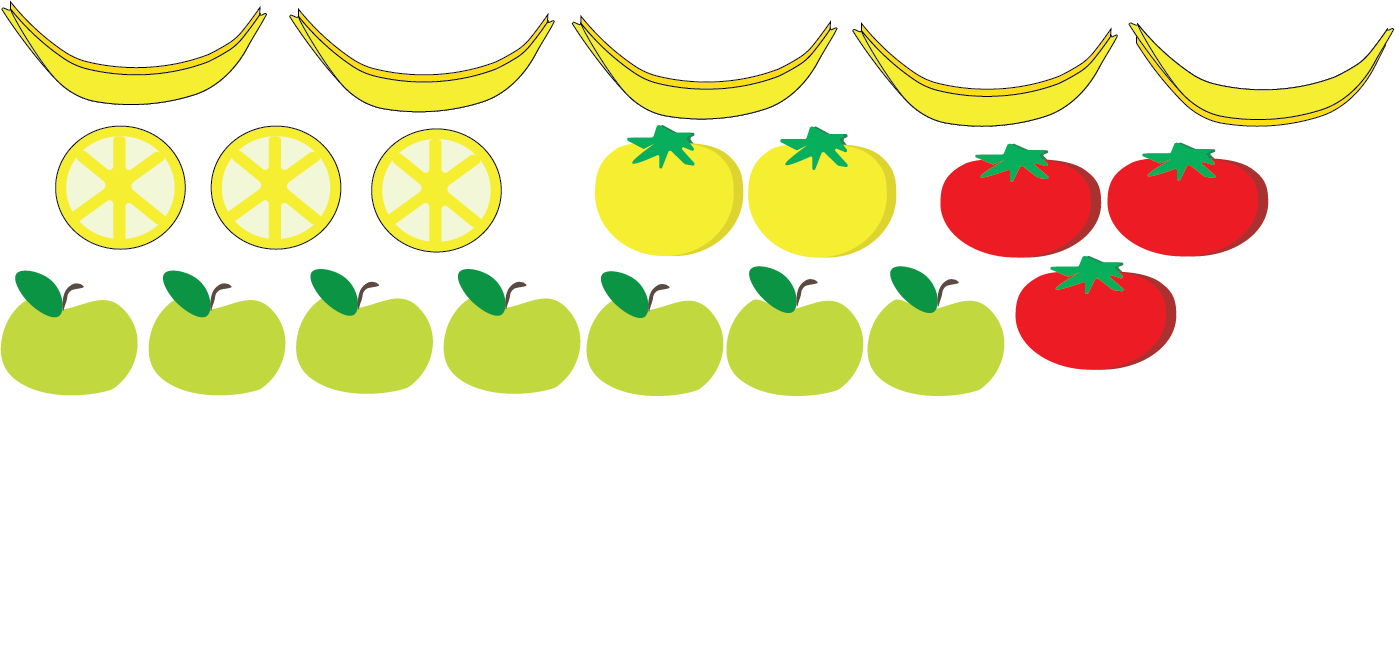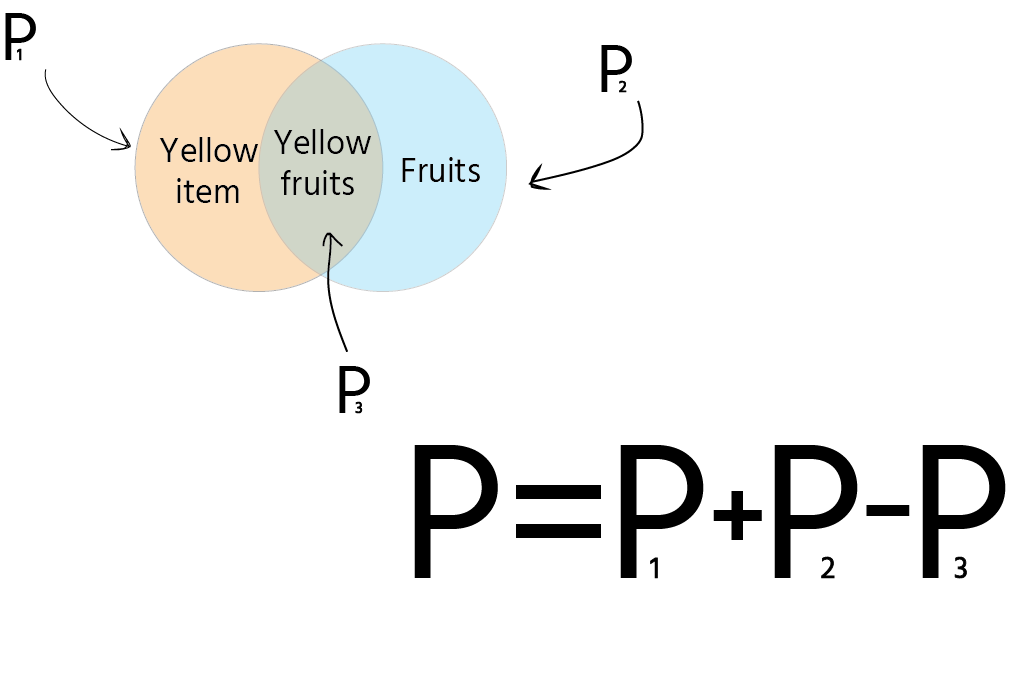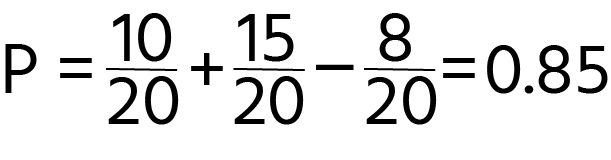Course Content

Probability TheoryCalculate Connected Probabilities

It seems to me that everything was pretty simple in the previous chapter. If we wanted to calculate the probability of getting success for either one or second event, we just added them together. But let's move to an interesting example.

Example:

Imagine that we have `5` bananas, `3` lemons, `2` yellow tomatoes, `3` red tomatoes, and `7` green apples. Calculate the probability of getting a fruit or yellow item.As you may recognize, fruit can be a yellow item too, so this formula is going to be a little bit complicated.Explanation:

The yellow circle defines all yellow items (yellow tomato, lemon, banana), and the blue circle represents all fruits (bananas, lemon, apple). But fruit can be yellow(banana, lemon). We can see the intersection of this too circle, which means that if we just add two probabilities, we will calculate yellow fruits twice, so it is crucial to subtract the probability of getting yellow fruit.

Solution: